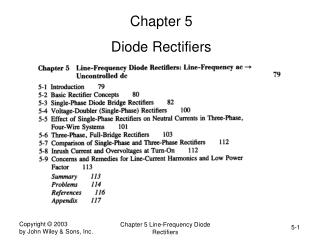DownloadDownload PresentationChapter 5 Diode Rectifiers

# Chapter 5 Diode Rectifiers

Download Presentation## Chapter 5 Diode Rectifiers

- - - - - - - - - - - - - - - - - - - - - - - - - - - E N D - - - - - - - - - - - - - - - - - - - - - - - - - - -
##### Presentation Transcript

1. Chapter 5 Diode Rectifiers Chapter 5 Line-Frequency Diode Rectifiers

2. Diode Rectifier Block Diagram • Uncontrolled utility interface (ac to dc) Chapter 5 Line-Frequency Diode Rectifiers

3. A Simple Circuit • Resistive load Chapter 5 Line-Frequency Diode Rectifiers

4. A Simple Circuit (R-L Load) • Current continues to flows for a while even after the input voltage has gone negative Chapter 5 Line-Frequency Diode Rectifiers

5. A Simple Circuit (Load has a dc back-emf) • Current begins to flow when the input voltage exceeds the dc back-emf • Current continues to flows for a while even after the input voltage has gone below the dc back-emf Chapter 5 Line-Frequency Diode Rectifiers

6. Single-Phase Diode Rectifier Bridge • Large capacitor at the dc output for filtering and energy storage Chapter 5 Line-Frequency Diode Rectifiers

7. Diode-Rectifier Bridge Analysis • Two simple (idealized) cases to begin with Chapter 5 Line-Frequency Diode Rectifiers

8. Redrawing Diode-Rectifier Bridge • Two groups, each with two diodes Chapter 5 Line-Frequency Diode Rectifiers

9. Waveforms with a purely resistive load and a purely dc current at the output • In both cases, the dc-side voltage waveform is the same Chapter 5 Line-Frequency Diode Rectifiers

10. Diode-Rectifier Bridge Input Current • Idealized case with a purely dc output current Chapter 5 Line-Frequency Diode Rectifiers

11. Diode-Rectifier Bridge Analysis with AC-Side Inductance • Output current is assumed to be purely dc Chapter 5 Line-Frequency Diode Rectifiers

12. Understanding Current Commutation • Assuming inductance in this circuit to be zero Chapter 5 Line-Frequency Diode Rectifiers

13. Understanding Current Commutation (cont.) • Inductance in this circuit is included Chapter 5 Line-Frequency Diode Rectifiers

14. Current Commutation Waveforms • Shows the volt-seconds needed to commutate current Chapter 5 Line-Frequency Diode Rectifiers

15. Current Commutation in Full-Bridge Rectifier • Shows the necessary volt-seconds Chapter 5 Line-Frequency Diode Rectifiers

16. Understanding Current Commutation • Note the current loops for analysis Chapter 5 Line-Frequency Diode Rectifiers

17. Rectifier with a dc-side voltage Chapter 5 Line-Frequency Diode Rectifiers

18. DC-Side Voltage and Current Relationship • Zero current corresponds to dc voltage equal to the peak of the input ac voltage Chapter 5 Line-Frequency Diode Rectifiers

19. Effect of DC-Side Current on THD, PF and DPF • Very high THD at low current values Chapter 5 Line-Frequency Diode Rectifiers

20. Crest Factor versus the Current Loading • The Crest Factor is very high at low values of current Chapter 5 Line-Frequency Diode Rectifiers

21. Diode-Rectifier with a Capacitor Filter • Power electronics load is represented by an equivalent load resistance Chapter 5 Line-Frequency Diode Rectifiers

22. Diode Rectifier Bridge • Equivalent circuit for analysis on one-half cycle basis Chapter 5 Line-Frequency Diode Rectifiers

23. Diode-Bridge Rectifier: Waveforms • Analysis using MATLAB Chapter 5 Line-Frequency Diode Rectifiers

24. Diode-Bridge Rectifier: Waveforms • Analysis using PSpice Chapter 5 Line-Frequency Diode Rectifiers

25. Input Line-Current Distortion • Analysis using PSpice Chapter 5 Line-Frequency Diode Rectifiers

26. Line-Voltage Distortion • PCC is the point of common coupling Chapter 5 Line-Frequency Diode Rectifiers

27. Line-Voltage Distortion • Distortion in voltage supplied to other loads Chapter 5 Line-Frequency Diode Rectifiers

28. Voltage Doubler Rectifier • In 115-V position, one capacitor at-a-time is charged from the input. Chapter 5 Line-Frequency Diode Rectifiers

29. A Three-Phase, Four-Wire System • A common neutral wire is assumed Chapter 5 Line-Frequency Diode Rectifiers

30. Current in A Three-Phase, Four-Wire System • The current in the neutral wire can be very high Chapter 5 Line-Frequency Diode Rectifiers

31. Three-Phase, Full-Bridge Rectifier • Commonly used Chapter 5 Line-Frequency Diode Rectifiers

32. Three-Phase, Full-Bridge Rectifier: Redrawn • Two groups with three diodes each Chapter 5 Line-Frequency Diode Rectifiers

33. Three-Phase, Full-Bridge Rectifier Waveforms • Output current is assumed to be dc Chapter 5 Line-Frequency Diode Rectifiers

34. Three-Phase, Full-Bridge Rectifier: Input Line-Current • Assuming output current to be purely dc and zero ac-side inductance Chapter 5 Line-Frequency Diode Rectifiers

35. Three-Phase, Full-Bridge Rectifier • Including the ac-side inductance Chapter 5 Line-Frequency Diode Rectifiers

36. 3-Phase Rectifier: Current Commutation • output current is assumed to be purely dc Chapter 5 Line-Frequency Diode Rectifiers

37. Rectifier with a Large Filter Capacitor • Output voltage is assumed to be purely dc Chapter 5 Line-Frequency Diode Rectifiers

38. Three-Phase, Full-Bridge Rectifier • THD, PF and DPF as functions of load current Chapter 5 Line-Frequency Diode Rectifiers

39. Crest Factor versus the Current Loading • The Crest Factor is very high at low values of current Chapter 5 Line-Frequency Diode Rectifiers

40. Three-Phase Rectifier Waveforms • PSpice-based analysis Chapter 5 Line-Frequency Diode Rectifiers